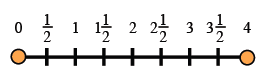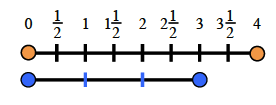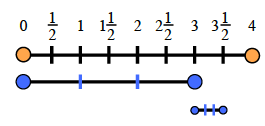### Home > CC1MN > Chapter 6 > Lesson 6.3.2 > Problem6-81

6-81.

Additional Challenge: Jill lives $3\frac { 1 } { 2 }$ miles from school. One morning, her friend was giving her a ride. When they were $\frac { 2 } { 3 }$ of the way to school, their car broke down and they had to walk the rest of the way. Draw a picture to help you figure out how far they walked.

If Jill and her friend were able to drive $\frac{2}{3}$ of the way to school, then they had to walk $\frac{1}{3}$ of the way.

The number line below is numbered $0$ through $4$. In order to find the total value of $\frac{1}{3}$ of $3\frac{1}{2}$,  A number line, scaled and labeled for each half, from 0 to 4.

First, find $\frac{1}{3}$ or $3$.  A line segment is added below the number line, divided in thirds. The sections are aligned with the numbers 0, 1, 2, and 3.

Now find $\frac{1}{3}$ of $\frac{1}{2}$A line segment is added below both line segments, also divided in thirds. The first mark is aligned with 3, and the last mark, with 3 and 1 half.

Were you able to find the distance Jill walked?
Remember to draw a picture of your own!